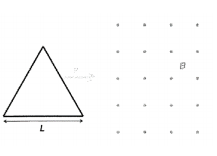Problem: An equilateral triangular loop moves into a 0.50 T magnetic field with a constant speed of v = 5 m/s as shown. The loop has sides of length L = 0.50 m, a total resistance of 0.10 Ω, and it enters the field at t = 0 s. What is the direction of the induced current in the loop? Provide a complete justification for your answer.

FREE Expert Solution
90% (422 ratings)
Problem Details

An equilateral triangular loop moves into a 0.50 T magnetic field with a constant speed of v = 5 m/s as shown. The loop has sides of length L = 0.50 m, a total resistance of 0.10 Ω, and it enters the field at t = 0 s. What is the direction of the induced current in the loop? Provide a complete justification for your answer.